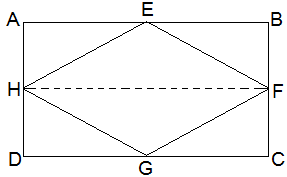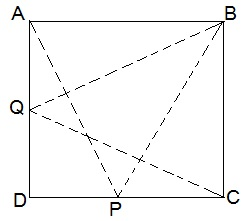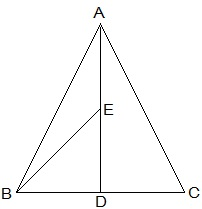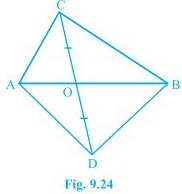# NCERT Solutions for Class 9th: Ch 9 Areas of Parallelograms and Triangles Maths (Part-1)

#### NCERT Solutions for Class 9th: Ch 9 Areas of Parallelograms and Triangles Maths (Part-1)

Page No: 155

Exercise 9.1

1. Which of the following figures lie on the same base and between the same parallels. In such a case, write the common base and the two parallels.(i) Trapezium ABCD and ΔPDC lie on the same DC and between the same parallel lines AB and DC.
(ii) Parallelogram PQRS and  trapezium SMNR lie on the same base SR but not between the same parallel lines.
(iii) Parallelogram PQRS and ΔRTQ lie on the same base QR and between the same parallel lines QR and PS.
(iv) Parallelogram ABCD and ΔPQR do not lie on the same base but between the same parallel lines BC and AD.
(v) Quadrilateral ABQD and trapezium APCD lie on the same base AD and between the same parallel lines AD and BQ.
(vi) Parallelogram PQRS and parallelogram ABCD do not lie on the same base SR but between the same parallel lines SR and PQ.

Page No: 159

Exercise 9.2

1. In Fig. 9.15, ABCD is a parallelogram, AE ⊥ DC and CF ⊥ AD. If AB = 16 cm, AE = 8 cm and CF = 10 cm, find AD.Given,
AB = CD = 16 cm (Opposite sides of a parallelogram)
CF = 10 cm and AE = 8 cm
Now,
Area of parallelogram = Base × Altitude
= CD × AE = AD × CF
⇒ 16 × 8 = AD × 10

2. If E,F,G and H are respectively the mid-points of the sides of a parallelogram ABCD, show that
ar (EFGH) = 1/2 ar(ABCD).Given,
E,F,G and H are respectively the mid-points of the sides of a parallelogram ABCD.
To Prove,
ar (EFGH) = 1/2 ar(ABCD)
Construction,
H and F are joined.
Proof,
AD || BC and AD = BC (Opposite sides of a parallelogram)
⇒ 1/2 AD = 1/2 BC
Also,
AH || BF and and DH || CF
⇒ AH = BF and DH = CF (H and F are mid points)
Thus, ABFH and HFCD are parallelograms.
Now,
ΔEFH and ||gm ABFH lie on the same base FH and between the same parallel lines AB and HF.
∴ area of EFH = 1/2 area of ABFH --- (i)
also, area of GHF = 1/2 area of HFCD --- (ii)
area of ΔEFH + area of ΔGHF = 1/2 area of ABFH + 1/2 area of HFCD
⇒ area of EFGH = area of ABFH
⇒ ar (EFGH) = 1/2 ar(ABCD)

3. P and Q are any two points lying on the sides DC and AD respectively of a parallelogram ABCD. Show that ar(APB) = ar(BQC).ΔAPB and ||gm ABCD are on the same base AB and between same parallel AB and DC.
Therefore,
ar(ΔAPB) = 1/2 ar(||gm ABCD) --- (i)
Similarly,
ar(ΔBQC) = 1/2 ar(||gm ABCD) --- (ii)
From (i) and (ii),
we have ar(ΔAPB) = ar(ΔBQC)

4. In Fig. 9.16, P is a point in the interior of a parallelogram ABCD. Show that
(i) ar(APB) + ar(PCD) = 1/2 ar(ABCD)
(ii) ar(APD) + ar(PBC) = ar(APB) + ar(PCD)
[Hint : Through P, draw a line parallel to AB.](i) A line GH is drawn parallel to AB passing through P.
In a parallelogram,
AB || GH (by construction) --- (i)
Thus,
AD || BC ⇒ AG || BH --- (ii)
From equations (i) and (ii),
ABHG is a parallelogram.
Now,
In ΔAPB and parallelogram ABHG are lying on the same base AB and between the same parallel lines AB and GH.
∴ ar(ΔAPB) = 1/2 ar(ABHG) --- (iii)
also,
In ΔPCD and parallelogram CDGH are lying on the same base CD and between the same parallel lines CD and GH.
∴ ar(ΔPCD) = 1/2 ar(CDGH) --- (iv)
ar(ΔAPB) + ar(ΔPCD) = 1/2 {ar(ABHG) + ar(CDGH)}
⇒ ar(APB) + ar(PCD) = 1/2 ar(ABCD)

(ii) A line EF is drawn parallel to AD passing through P.
In a parallelogram,
AD || EF (by construction) --- (i)
Thus,
AB || CD ⇒ AE || DF --- (ii)
From equations (i) and (ii),
AEDF is a parallelogram.
Now,
In ΔAPD and parallelogram AEFD are lying on the same base AD and between the same parallel lines AD and EF.
∴ ar(ΔAPD) = 1/2 ar(AEFD) --- (iii)
also,
In ΔPBC and parallelogram BCFE are lying on the same base BC and between the same parallel lines BC and EF.
∴ ar(ΔPBC) = 1/2 ar(BCFE) --- (iv)
ar(ΔAPD) + ar(ΔPBC) = 1/2 {ar(AEFD) + ar(BCFE)}
⇒ ar(APD) + ar(PBC) = ar(APB) + ar(PCD)

5. In Fig. 9.17, PQRS and ABRS are parallelograms and X is any point on side BR. Show that
(i) ar (PQRS) = ar (ABRS)
(ii) ar (AXS) = 1/2 ar (PQRS)(i) Parallelogram PQRS and ABRS lie on the same base SR and between the same parallel lines SR and PB.
∴ ar(PQRS) = ar(ABRS) --- (i)
(ii) In ΔAXS and parallelogram ABRS are lying on the same base AS and between the same parallel lines AS and BR.
∴ ar(ΔAXS) = 1/2 ar(ABRS) --- (ii)
From (i) and (ii),
ar(ΔAXS) = 1/2 ar(PQRS)

Page No: 106

6. A farmer was having a field in the form of a parallelogram PQRS. She took any point A on RS and joined it to points P and Q. In how many parts the fields is divided? What are the shapes of these parts? The farmer wants to sow wheat and pulses in equal portions of the field separately. How should she do it?The field is divided into three parts. The three parts are in the shape of triangle. ΔPSA, ΔPAQ and ΔQAR.
Area of ΔPSA + ΔPAQ + ΔQAR = Area of PQRS --- (i)
Area of ΔPAQ = 1/2 area of PQRS --- (ii)
Triangle and parallelogram on the same base and between the same parallel lines.
From (i) and (ii),
Area of ΔPSA + Area of ΔQAR = 1/2 area of PQRS --- (iii)
Clearly from (ii) and (iii),
Farmer must sow wheat or pulses in ΔPAQ or either in both ΔPSA and ΔQAR.

Page No: 162

Exercise 9.3

1. In Fig.9.23, E is any point on median AD of a ΔABC. Show that ar (ABE) = ar(ACE).Given,
AD is median of ΔABC. Thus, it will divide ΔABC into two triangles of equal area.
∴ ar(ABD) = ar(ACD) --- (i)
also,
ED is the median of ΔABC.
∴ ar(EBD) = ar(ECD) --- (ii)
Subtracting (ii) from (i),
ar(ABD) - ar(EBD) = ar(ACD) - ar(ECD)
⇒ ar(ABE) = ar(ACE)

2. In a triangle ABC, E is the mid-point of median AD. Show that ar(BED) = 1/4 ar(ABC).ar(BED) = (1/2) × BD × DE
As E is the mid-point of AD,
Thus, AE = DE
As AD is the median on side BC of triangle ABC,
Thus, BD = DC
Therefore,
BD = (1/2)BC --- (ii)
From (i) and (ii),
ar(BED) = (1/2) × (1/2) BC × (1/2)AD ⇒ ar(BED) = (1/2) × (1/2) ar(ABC)
⇒ ar(BED) = 1/4 ar(ABC)

3. Show that the diagonals of a parallelogram divide it into four triangles of equal area.O is the mid point of AC and BD. (diagonals of bisect each other)
In ΔABC, BO is the median.
∴ ar(AOB) = ar(BOC) --- (i)
also,
In ΔBCD, CO is the median.
∴ ar(BOC) = ar(COD) --- (ii)
In ΔACD, OD is the median.
∴ ar(AOD) = ar(COD) --- (iii)
In ΔABD, AO is the median.
∴ ar(AOD) = ar(AOB) --- (iv)
From equations (i), (ii), (iii) and (iv),
ar(BOC) = ar(COD) = ar(AOD) = ar(AOB)
So, the diagonals of a parallelogram divide it into four triangles of equal area.

4. In Fig. 9.24, ABC and ABD are two triangles on the same base AB. If line- segment CD is bisected by AB at O, show that:
ar(ABC) = ar(ABD).